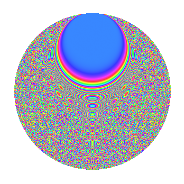# Properties

 Label 2100.2.dkLevel 2100 Weight 2 Character orbit dk Rep. character $$\chi_{2100}(53,\cdot)$$ Character field $$\Q(\zeta_{60})$$ Dimension 1280 Sturm bound 960

# Related objects

## Defining parameters

 Level: $$N$$ $$=$$ $$2100 = 2^{2} \cdot 3 \cdot 5^{2} \cdot 7$$ Weight: $$k$$ $$=$$ $$2$$ Character orbit: $$[\chi]$$ $$=$$ 2100.dk (of order $$60$$ and degree $$16$$) Character conductor: $$\operatorname{cond}(\chi)$$ $$=$$ $$525$$ Character field: $$\Q(\zeta_{60})$$ Sturm bound: $$960$$

## Dimensions

The following table gives the dimensions of various subspaces of $$M_{2}(2100, [\chi])$$.

Total New Old
Modular forms 7872 1280 6592
Cusp forms 7488 1280 6208
Eisenstein series 384 0 384

## Trace form

 $$1280q - 4q^{7} + O(q^{10})$$ $$1280q - 4q^{7} - 12q^{15} + 8q^{25} - 10q^{33} + 8q^{37} + 40q^{39} - 32q^{43} - 70q^{45} + 16q^{55} + 100q^{57} + 24q^{63} + 16q^{67} + 140q^{69} + 8q^{73} - 40q^{75} + 40q^{85} + 38q^{87} + 6q^{93} + 80q^{97} + O(q^{100})$$

## Decomposition of $$S_{2}^{\mathrm{new}}(2100, [\chi])$$ into newform subspaces

The newforms in this space have not yet been added to the LMFDB.

## Decomposition of $$S_{2}^{\mathrm{old}}(2100, [\chi])$$ into lower level spaces

$$S_{2}^{\mathrm{old}}(2100, [\chi]) \cong$$ $$S_{2}^{\mathrm{new}}(525, [\chi])$$$$^{\oplus 3}$$$$\oplus$$$$S_{2}^{\mathrm{new}}(1050, [\chi])$$$$^{\oplus 2}$$

## Hecke characteristic polynomials

There are no characteristic polynomials of Hecke operators in the database